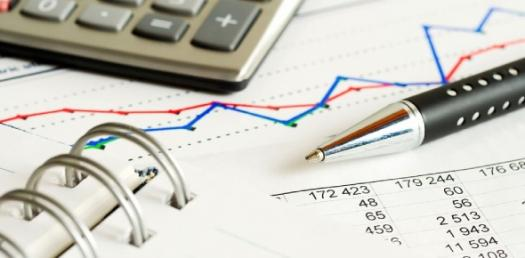# Accounting 1 (Summer 2018)

30 Questions | Total Attempts: 119SettingsHello Class! Congrats for making it to this point. Please give this your best shot! Good Bless!

• 1.
If adjusting entries are recorded in the worksheet, there is no need for them to be journalized or posted
• A.

True

• B.

False

• 2.
Accrual accounting involves all of the following except
• A.

Recording all revenues when cash was received

• B.

Applying the matching rule

• C.

Recognizing expense when incurred

• D.

• E.

All of the above

• 3.
Is it true that the trial balance totals should agree?
• A.

No, there are sometimes good reasons why they differ

• B.

Yes, except where the trial balance is extracted at the year end

• C.

Yes, always

• D.

No, because it is not a balance sheet

• 4.
The current portion of a long term debt should
• A.

Be classsified as a long term liability

• B.

Not be separated from the long term portion of debt

• C.

Be paid immediately

• D.

Be reclassified as a current liability

• 5.
Which of the following accounts is what is known as a temporary or nominal account ?
• A.

Asset

• B.

Owner's Equity (Capital)

• C.

Liability

• D.

Revenue

• 6.
A Debit:
• A.

Increase an Asset Account

• B.

Decrease an Asset Account

• C.

Increase an Liability Account

• D.

Increase owner's equity

• 7.
Under accrual accounting, revenue is recorded
• A.

When the cash is collected, regardless of when the services are performed

• B.

When the services are performed, regardless of when the cash is received

• C.

• 8.
• A.

Not needed under the accrual basis of accounting

• B.

Prepared at the option of the accountant

• C.

Prepared at the beginning of the accounting period to update all accounts.

• D.

Prepared at the end of the accounting period to update certain accounts.

• 9.
Prepaid insurance is reported on the balance sheet as a(n):
• A.

Expense

• B.

Liability

• C.

Asset

• D.

Contra asset

• 10.
The book value of a plant asset is the
• A.

Accumulated depreciation less the cost of the asset

• B.

Cost of the asset

• C.

Balance in the accumulated depreciation account

• D.

Cost of the asset less the accumulated depreciation

• 11.
A liability that arises from an expense that has not yet been paid is a(n):
• A.

Unearned expense

• B.

Prepaid expense

• C.

Accrued expense

• D.

Accrued revenue

• 12.
The financial statements are prepared from the
• A.

• B.

• C.

Ledger

• D.

• 13.
According to the revenue principle, revenue should be recorded
• A.

Before it has been earned

• B.

• C.

When it has been earned

• D.

Whenever the company needs to record the revenue

• 14.
An expense that is paid in advance is a(n):
• A.

Unearned expense

• B.

Prepaid expense

• C.

Liability

• D.

Unearned asset

• 15.
Current assets include
• A.

Cash and receivables

• B.

Cash and payables

• C.

Land and Building

• D.

Retained earnings

• 16.
Which account is debited in the adjusting entry to record depreciation expense during the current period?
• A.

Accumulated Depreciation

• B.

Equipment

• C.

Depreciation Expense

• D.

Depreciation Payable

• 17.
Which of the following accurately describes the account type of the Accumulated Depreciation account?
• A.

Contra-expense account

• B.

Liability account

• C.

Contra-asset account

• D.

Expense account

• 18.
The Accounting Equation is: ASSETS  =  LIABILITIES + OWNER'S EQUITY  OR ASSETS - LIABILITIES = OWNER'S EQUITY
• A.

True

• B.

False

• 19.
Which of the following is/are a purpose of adjusting entries?
• A.

To update the accounts in the books

• B.

To apply the matching principle

• C.

To properly reflect the the correct net income

• D.

To make the equation A=L+C more accurate

• E.

All of the above

• 20.
Which one of the following categories of account is "credited" when it is increased?
• A.

Revenue

• B.

Purchases

• C.

Asset

• D.

Expenses

• 21.
Which one of the following categories of account is "debited" when it is increased?
• A.

Revenue

• B.

Purchases

• C.

Sources of fund

• D.

Liability

• 22.
A company using the accrual basis of accounting pays P15,000 for a television advertising campaign.  Commercials will run evenly in December, January, and February. How much expense will be reported on an income statement prepared for the month of December?
• A.

P0

• B.

P5,000

• C.

P10,000

• D.

P15,000

• 23.
A business sells merchandise to the customer for P85. The merchandise cost is P65. For this sale, how much is the Gross Profit?
• A.

P65

• B.

P20

• C.

Neither amount

• 24.
The book value of an asset that cost P20,000 and has accumulated depreciation of P6,000 is
• A.

P20,000

• B.

P 6,000

• C.

P26,000

• D.

P14,000

• 25.
A company has P800 beginning balance of Supplies (asset). At the end of the month, the Supplies on hand is P150.  The adjusting entry for this company is:
• A.

Debit supplies of P150 and a credit of P150 to Supplies Expense

• B.

Debit supplies Expense of P150 and a credit of P150 to Supplies

• C.

Debit supplies Expense of P650 and a credit of P650 to Supplies

• D.

There is not enough information given to prepare the entry

Related TopicsBack to top